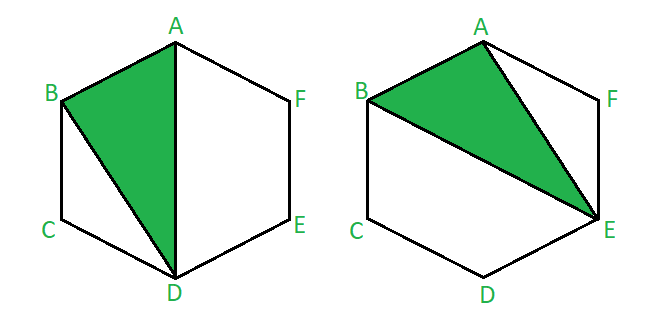# Number of triangles formed by joining vertices of n-sided polygon with one side common

Given N-sided polygon we need to find the number of triangles formed by joining the vertices of the given polygon with exactly one side being common.

Examples:

Input : 6
Output : 12
The image below is of a triangle forming inside a Hexagon by joining vertices as shown above. The two triangles formed has one side (AB) common with that of a polygon.It depicts that with one edge of a hexagon we can make two triangles with one side common. We can’t take C or F as our third vertex as it will make 2 sides common with the hexagon.Triangle with one side common of a hexagon

No of triangles formed ie equal to 12 as there are 6 edges in a hexagon.

Input : 5
Output : 5

## Recommended: Please try your approach on {IDE} first, before moving on to the solution.

Approach :

• To make a triangle with one side common with a polygon the two vertices adjacent to the chosen common vertices cannot be considered as the third vertex of a triangle.
• First select any one edge from the polygon. Consider this edge to be the common edge. Number of ways to select an edge in a polygon would be equal to n.
• Now ,to form a triangle ,select any of the (n-4) vertices left .Two vertices of the common edge and two vertices adjacent to the common edge cannot be considered.
• Number of triangle formed by joining the vertices of an n-sided polygon with one side common would be equal to n * ( n – 4) .

Below is the implementation of the above approach:

## C++

 `// C++ program to implement ` `// the above problem ` `#include ` `using` `namespace` `std; ` ` `  `// Function to find the number of triangles ` `void` `findTriangles(``int` `n) ` `{ ` `    ``int` `num; ` `    ``num = n * (n - 4); ` ` `  `    ``// print the number of triangles ` `    ``cout << num; ` `} ` ` `  `// Driver code ` `int` `main() ` `{ ` `    ``// initialize the number of sides of a polygon ` `    ``int` `n; ` `    ``n = 6; ` ` `  `    ``findTriangles(n); ` ` `  `    ``return` `0; ` `} `

## Java

 `// Java program to implement ` `// the above problem ` `class` `GFG ` `{ ` `     `  `// Function to find the number of triangles ` `static` `void` `findTriangles(``int` `n) ` `{ ` `    ``int` `num; ` `    ``num = n * (n - ``4``); ` ` `  `    ``// print the number of triangles ` `    ``System.out.println(num); ` `} ` ` `  `// Driver code ` `public` `static` `void` `main(String [] args) ` `{ ` `    ``// initialize the number of sides of a polygon ` `    ``int` `n; ` `    ``n = ``6``; ` ` `  `    ``findTriangles(n); ` ` `  `} ` `} ` ` `  `// This code is contributed by ihritik `

## Python3

 `# Python3 program to implement ` `# the above problem ` ` `  `# Function to find the number of triangles ` `def` `findTriangles(n): ` `    ``num ``=` `n ``*` `(n ``-` `4``) ` ` `  `    ``# print the number of triangles ` `    ``print``(num) ` ` `  `# Driver code  ` `# initialize the number of sides of a polygon ` `n ``=` `6` ` `  `findTriangles(n) ` ` `  `# This codee is contributed by mohit kumar 29 `

## C#

 `// C# program to implement ` `// the above problem ` `using` `System; ` ` `  `class` `GFG ` `{ ` `     `  `// Function to find the number of triangles ` `static` `void` `findTriangles(``int` `n) ` `{ ` `    ``int` `num; ` `    ``num = n * (n - 4); ` ` `  `    ``// print the number of triangles ` `    ``Console.WriteLine(num); ` `} ` ` `  `// Driver code ` `public` `static` `void` `Main() ` `{ ` `    ``// initialize the number of sides of a polygon ` `    ``int` `n; ` `    ``n = 6; ` ` `  `    ``findTriangles(n); ` ` `  `} ` `} ` ` `  `// This code is contributed by ihritik `

Output:

```12
```

Time Complexity: O(1)

Attention reader! Don’t stop learning now. Get hold of all the important DSA concepts with the DSA Self Paced Course at a student-friendly price and become industry ready.

My Personal Notes arrow_drop_upCheck out this Author's contributed articles.

If you like GeeksforGeeks and would like to contribute, you can also write an article using contribute.geeksforgeeks.org or mail your article to contribute@geeksforgeeks.org. See your article appearing on the GeeksforGeeks main page and help other Geeks.

Please Improve this article if you find anything incorrect by clicking on the "Improve Article" button below.